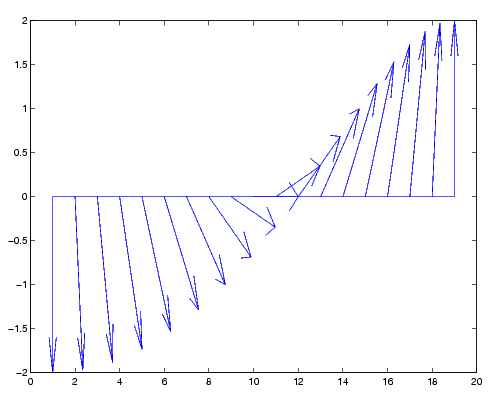MATLAB Function Referencefeather

Plot velocity vectors

Syntax

• ```feather(U,V)
feather(Z)
feather(...,`LineSpec`)
feather(axes_handle,...)
h = feather(...)
```

Description

A feather plot displays vectors emanating from equally spaced points along a horizontal axis. You express the vector components relative to the origin of the respective vector.

```feather(U,V) ``` displays the vectors specified by `U` and `V`, where `U` contains the x components as relative coordinates, and `V` contains the y components as relative coordinates.

```feather(Z) ``` displays the vectors specified by the complex numbers in `Z`. This is equivalent to `feather(real(Z),imag(Z))`.

```feather(...,LineSpec) ``` draws a feather plot using the line type, marker symbol, and color specified by `LineSpec`.

```feather(axes_handle,...) ``` plots into the axes with handle `axes_handle` instead of the current axes (`gca`).

```h = feather(...) ``` returns the handles to line objects in `h`.

Examples

Create a feather plot showing the direction of `theta`.

• ```theta = (-90:10:90)`*`pi/180;
r = 2`*`ones(size(theta));
[u,v] = pol2cart(theta,r);
feather(u,v);```

See Also

`compass`, `LineSpec`, `rose`

Direction and Velocity Plots for related functions

© 1994-2005 The MathWorks, Inc.